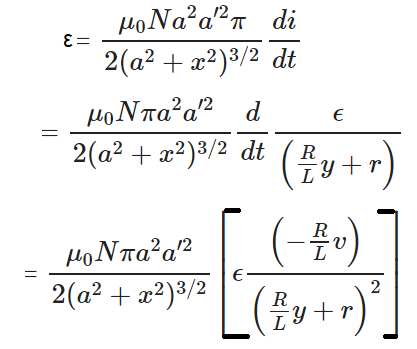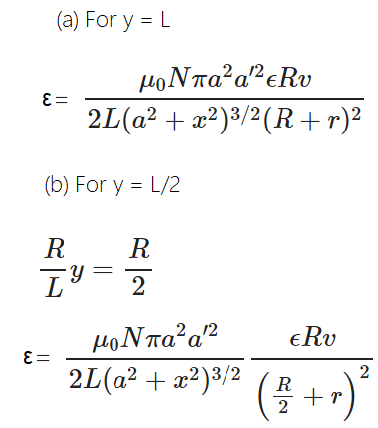# HC Verma Solutions Class 12 Chapter 16 Electromagnetic Induction

HC Verma Solutions Class 12 Electromagnetic Induction chapter 16 contains solved examples and detailed answers to questions given in this unit. Students can refer the solutions and develop gain better efficiency in tackling complex and difficult questions that students might come across while learning the different concepts.

In order to make studying easier, the HC Verma Solutions provided here offers the right knowledge that will further help them be proficient with the terminologies and concepts. In essences, students will be able to solve problems effectively and perform well in exams like IIT JEE amongst others.

The chapter includes some important topics likes;

• Lenz’s Law
• Induced emf
• Eddy Current
• Self Induction
• Growth and Decay of Current
• Energy Stored in an Inductor
• Mutual Induction
• Induction Coil

## Class 12 Important Questions In Chapter 16

1. Do you think that an emf is induced in the metallic loop when it is placed in a nonuniform magnetic field?

2. In one instance we take an inductor and connect it to a battery through a switch. Can you explain why the emf induced in the inductor is much larger when the switch is opened as compared when it is closed?

3. Which is greater, the energy density in a solenoid at its centre or that near its ends?

4. What will happen to the bar magnet after it is released from rest along the axis of a very long, vertical copper tube?

(a) It will gain acceleration g (b) It will oscillate (c) It will come to a stop in the tube (d) It will move with constant speed

5. A circuit LR is connected with a battery at t = 0. Name the quantities which will not be zero after the connection is made?

(a) the power delivered by the battery (b)  emf induced in the inductor (c) the current in the circuit (d) magnetic field energy in the inductor

## HC Verma Solutions Vol 2 Electromagnetic Induction- Chapter 16

Question 1: Calculate the dimension of

$(a) \int \vec E . \vec{dl} \; \;(b) v Bl \; \; (c) \frac{d \phi_B}{dt}$

The symbols have their usual meanings.

Solution:

$(a) \int \vec E . \vec{dl} = \epsilon$

ε = emf of voltage

$\int \vec E . \vec{dl}$ has dimensions of voltage.

We know, voltage, V = W/Q

W = work done and Q = Charge

Now,

Dimensions of W = [ML2T-2]

Dimensions of Q = [AT]

So, Dimensions of V = [ML2T-3A-1]

(b) vBl is the motional emf developed due to motion of conductor. so it has same dimensions as of voltage.

From part (a)

Dimensions of vBl = [ML2T-3A-1]

(c) We know, ε = -dφ/dt

ε has same dimensions as of emf.

So, dimensions of dφB/dt = [ML2T-3A-1]

Question 2: The flux of magnetic field through a closed conducing φ = at2 + bt + c.

(a) Writer the S.I. units of a, b and c.

(b) If the magnitudes of a, b, and c are 0.20, 0.40 and 0.60 respectively, find the induced emf at t = 2s.

Solution:

(a)

Dimensions of φ, at2, bt and c are same

Units of a = φ/t2 = (φ/t)/t

We know, ε = -dφ/dt

Therefore, dimensions of φ/t is same as voltage.

So, S.I. units of a = volt/sec

Now, S.I. unit of b = φ/t = Volt

Here c = φ

S.I. unit of c = [φ] = Weber

(b) By faraday’s law of electromagnetic induction

ε = -dφ/dt

From given, ε = -d/dt [at2 + bt + c] = -(2at + b)

Putting the values of a, b and t, we have

ε = 2 x 0.2 x 2 + 0.4 = 1.2 Volt

Question 3: (a) The magnetic field in a region varies as shown in figure. Calculate the average induce emf in a conducting loop of area 2.0 × 10–3 m2 placed perpendicular to the field in each of the 10 ms intervals shown.

(b) In which intervals is the emf not constant? Neglect the behaviour near the ends of 10 ms intervals.

HC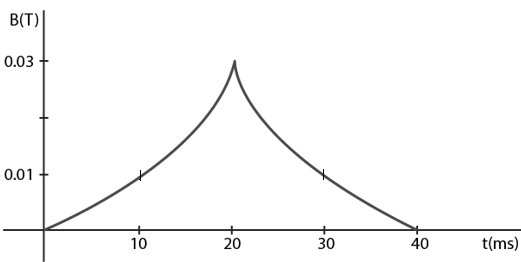Solution:

Area of loop = 2 x 10-3 m2 (given)

Magnetic flux at point A = φ = BA

[Since loop is placed perpendicular to the field]

The change in magnetic flux in 10 ms: Δφ = 2 x 10-5

So, the emf induced = ε = -Δφ/Δt

= – [2 x 10-5 – 0]/[10 x 10-3]

= -2 mV

The magnetic flux at point B = φ’ = BA

= 0.03 x 2 x 10-3

= 6 x 10-5

The change in magnetic flux in 10 ms: Δφ = 6 x 10-5 – 2 x 10-5

= 4 x 10-5

So, the emf induced = ε = -Δφ/Δt = – 4 mV

The magnetic flux at point C = φ” = BA

= 0.01 x 2 x 10-3

= 2 x 10-5

The change in magnetic flux in 10 ms: Δφ = 2 x 10-5 – 6 x 10-5

= -4 x 10-5

So, the emf induced = ε = -Δφ/Δt = 4 mV

The magnetic flux at point D = φ”’ = BA = 0

The change in magnetic flux in 10 ms: Δφ = 0 – 2 x 10-5

= 2 x 10-5

So, the emf induced = ε = -Δφ/Δt = 2 mV

(b) emf across the intervals:

0-10ms = -2mV

10-20ms = -4mV

20-30ms = 4mV

30-40ms = 2mV

emf is not constant in time intervals 10-20 ms and 20-30ms

Question 4: A conducting circular loop having a radius of 5.0 cm, is placed perpendicular to a magnetic field of 0.50 T. It is removed from the field in 0.50 s. Find the average emf produced in the loop during this time.

Solution:

Area of circular loop = A = πr2 = π(5 x 10-2)2

Initial magnetic flux through the loop: φ1 = BA coso = BA

[Since loop is placed perpendicular to magnetic field, so angle is zero degrees)

So, φ1 = BA = 0.5 x [π(5 x 10-2)2 ] = 125 π x 10-5

After loop is removed from the field after time Δt = 0.50s, the magnetic flux, φ2 , will be zero.

We know, Average induced emf in time interval Δt :

ε = [ φ1 – φ2]/t = [125 π x 10-5] / [5×10-1]

= 7.8 x 10-3 V

[Use π = 3.14]

Question 5: A conducting circular loop of area 1 mm2 is placed co-planarly with a long, straight wire at a distance of 20 cm from it. The straight wire carries as electric current which changes from 10 A to zero in 0.1 s. Find the average emf induced in the loop in 0.1 s.

Solution:

Area of circular loop = A = 1 mm2 = 1×10-6 m2

Separation between wire and loop = d = 20 cm = 20 x 10-2 m

Current through the wire = i = 10 A

Time = dt = 0.1 sec

Average induced emf in time interval dt.

ε = dφ/dt = BA/dt

= μ0i/2πd x A/dt

= [4π x 10-7x10]/[2πx2x10-1] x 10-6/[1×10-1]

= 1 x 10-10 V

Question 6: A square-shaped copper coil has edges of length 50 cm and contains 50 turns. It is placed perpendicular to a 1.0 T magnetic field. It is removed from the magnetic field in 0.25 s and restored in its original place in the next 0.25 s. Find the magnitude of the average emf induced in the loop during

(a) its removal, (b) its restoration and (c) its motion.

Solution:

Magnetic flux due to magnetic field B through cross section area A is

$\phi = \vec B . \vec A$

(a) During removal

Initial magnetic flux through the loop: φ1 = B.A

Let φ1 flux across the cross section at time interval t1.

=> φ1 = B.A = 50 x 0.5 x 0.5 = 12.5 Tm2

Let φ2 flux across the cross section at time interval t2.

Final magnetic flux through the loop = φ2 = 0

Time interval = Δt = 0.25s

Now,

Magnitude of average emf during removal = ε = – [φ2 – φ1]/ Δt

= – [0-12.5]/0.25

= 50 V

(b) During restoration:

Initial magnetic flux through the loop = φ1 = 0

And Final magnetic flux through the loop = φ2 = BA

=> φ2 = 1 x 50 x 0.5 x 0.5 = 12.5 tesla-m2

Time interval = Δt = 0.25s

Now,

Magnitude of average emf during restoration = ε = – [φ2 – φ1]/ Δt

= – [12.5 – 0]/0.25

= – 50 V

(c) During the motion

Initial and final magnetic flux through the loop = 0

=> φ1 = φ2 = 0

Time interval = Δt = 0.25s + 0.25s = 0.50s

Now,

Magnitude of average emf during motion = ε = – [φ2 – φ1]/ Δt

= 0

Question 7: Suppose the resistance of the coil in the previous problem is 25 Ω. Assume that the coil moves with uniform velocity during its removal and restoration. Find the thermal energy developed in the coil during

(a) its removal

(b) its restoration and

(c) its motion.

Solution:

(a) emf induced in the loop = 50 V

=> ε = 50 V

So, the current flowing through the loop = i = ε/r

r = 25Ω (given)

=> i = 50/25 = 2 A

Thermal energy developed in the coil during its removal in Δt = 0.25s

=> H = i2 r Δt

= 4 x 25 x 0.25s

= 25 J

(b)

emf induced in the loop = 50 V

=> ε = 50 V

So, the current flowing through the loop = i = ε/r

=> i = 50/25 = 2 A

Thermal energy developed in the coil during its removal in Δt = 0.25s

=> H = i2 r Δt = 25 J

(c) Total thermal energy developed = energy developed during removal + energy developed during restoration

=> H = 25 + 25 = 50 J

Question 8: A conducting loop of area 5.0 cm2 is placed in a magnetic field which varies sinusoidally with time as B = B0 sin ωt where B0 = 0.20 T and ω = 300 s–1. The normal to the coil makes an angle of 60° with the field. Find

(a) the maximum emf induced in the coil,

(b) the emf induced at τ = (π/900) s and

(c) the emf induced at t = (π/600) s.

Solution: Magnetic flux due to magnetic field B through cross section area A

$\phi = \vec B . \vec A = BA cos 60^0$ [Angle of the magnetic field with normal to coil is θ = 60o ]

Magnetic field at time t is: B = Bo sin ωt = (0.2) sin (300t)

Emf induced in coil = ε = -dθ/dt = d/dt [BA cos θ]

= d/dt[(Bo sin ωt) x (5×10-4) x 1/2]

= (5/2) (0.2 x10-4) d/dt [sin ωt]

= (5/2) (0.2×10-4) ω cos ωt

= (5/2) (0.2×10-4) (300) cos ωt

=> ε = 15 x 10-3 cos ωt …(1)

(a) Since maximum value of cos ωt = 1

(1)=> maximum Emf induced in coil = 0.015 V

(b) Magnitude of induced emf at t = π/900 s

(1)=> ε = 15 x 10-3 cos (300 x π/900)

= 15 x 10-3 x ½

= 7.5 x 10-3 V

(c) Magnitude of induced emf at t = π/600 s

(1)=> ε = 15 x 10-3 cos (300 x π/600)

= 15 x 10-3 x 0

= 0 V

Question 9: Figure shows a conducting square loop placed parallel to the pole-faces of a ring magnet. The pole-faces have an area of 1 cm2 each and the field between the poles is 0.10 T. The wires making the loop are all outside the magnetic field. If the magnet is removed in 1.0 s, what is the average emf induced in the loop?Solution:

As magnetic field through the square loop is perpendicular to the loop.

Initial magnetic flux, $\phi = \vec B . \vec A$

= BA cos (0) = 10-1 x 10-4 x 1 = 10-5

Given: Area of loop = A = 10-4 m and B = 0.10 T

Now, the induced emf in B:

ε = – Δφ/Δt = [10-5-0] /1 = 10-5 = 10 μV

Question 10: A conducting square loop having edges of length 2.0 cm is rotated through 180° about a diagonal in 0.20 s. A magnetic field B exists in the region which is perpendicular to the loop in its initial position. If the average induced emf during the rotation is 20 mV, find the magnitude of the magnetic field

Solution:

Average induced emf in time interval Δt:

ε = – Δφ/Δt = -[φ2 – φ1]/ Δt …(1)

Where φ2 and φ1flux across the cross section at time intervals t1 and t2.

Here φ = BA cos θ

Initial flux through the coil = φ1 = BA

[Initially, angle between area vector and magnetic field is 0°]

When it is rotated by 180° flux passing through the coil, the angle will be 180o

So, φ2 = BA cos(180o) = -BA

=> ε = 2BA/ Δt

On substituting the values of ϵ, B and Δt, we get

2 x 10-2 = 2 x B x 4 x 10-4/0.2

=>B = 5 T

Question 11: A conducting loop of face-area A and resistance R is placed perpendicular to a magnetic field B. The loop is withdrawn completely from the field. Find the charge which flows through any cross-section of the wire in the process. Note that it is independent of the shape of the loop as well as the way it is withdrawn.

Solution:

Magnetic flux through the loop : φ = BA cosθ

θ is the angle between area vector and magnetic field.

We know, Average induced emf in time interval Δt :

ε = – Δφ/Δt = -[φ2 – φ1]/ Δt …..(1)

Since initially loop is perpendicular to the applied magnetic field hence initial flux, so θ = 0

=> φ1 = BA

When the loop is withdrawn from the field flux : φ2 = 0

(1)=> ε = BA/ Δt

Now, Current flowing in the loop is calculated as

i = ε/R = BA/RΔt

The charge “Q” is flowing through any cross-section of the wire is calculated as:

Q = iΔt = BA/R

Question 12: A long solenoid of radius 2 cm has 100 turns/cm and carries a current of 5 A. A coil of radius 1 cm having 100 turns and a total resistance of 20 Ω is placed inside the solenoid coaxially. The coil is connected to a galvanometer. If the current in the solenoid is reversed in direction, find the charge flown through the galvanometer.

Solution:

Magnetic field inside solenoid : B = μ0 ni = 4 π x 10-7 x10000 x 5

=> B = 20 π x 10-3 T

Where n = number of turns per unit length = 10000 turns per mtr

Magnetic flux(ϕ) through the coil = φ = BA cos θ

Here, θ is the angle between area vector and magnetic field

Since, magnetic field inside solenoid is perpendicular to the coil, so θ = 0 at initially flux through the coil.

=> Total flux linking the second coil = φ1 = BA

[Because, cos0o = 1]

=> φ1 = B x π r’2 x N

=> φ1 = (20 π x 10-3) x π x 10-4 x 100

Where r’ = Radius of second coil = 1 cm = 0.01 m and

N = No. of turns in the coil = 100

When the direction of the current is reverse, total flux through second coil:

=> φ2 = – B x π r’2 x N

=> φ2 = – [(20 π x 10-3) x π x 10-4 x 100]

Now, change in flux through the 2nd coil:

dφ = φ2 – φ1

= 2 x [(20 π x 10-3) x π x 10-4 x 100]

= 4 π x 10-4

Now,

ε = dφ/ dt = [4 π x 10-4]/dt

Again, we know i = ε/R = [4 π x 10-4]/20dt

[R = 20 Ω (given)]

The charge through galvanometer = q = i dt = [4 π x 10-4]/(20dt) x dt

=>q = 2 x 10-4 C

Question 13: Figure shown a metallic square frame of edge an in a vertical plane. A uniform magnetic field B exists in the space in a direction perpendicular to the plane of the figure. Two boys pull the opposite corners of the square to deform it into a rhombus. They start pulling the corners at t = 0 and displace the corners at a uniform speed u.

(a) Find the induced emf in the frame at the instant when the angles at these corners reduced to 60°.

(b) Find the induced current in the frame at this instant if the total resistance of the frame is R.

(c) Find the total charge which flows through a side of the frame by the time the square is deformed into a straight line.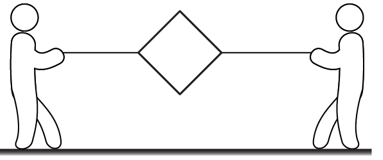Solution:

(a) when the angles at the corner reduce to 60°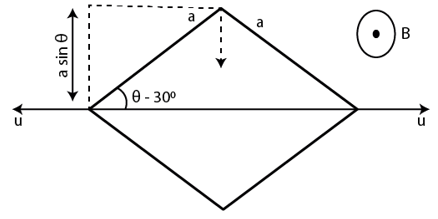The motional emf produced due to a conductor of length l moving with velocity v in magnetic field B is:

$\epsilon = (\vec v \times \vec B). \vec i$

The effective length of each side of frame:

leff = a sin 30o = a/2

[Effective length is the length perpendicular to velocity of corners.]

As velocity is perpendicular to magnetic field the equation, emf induced in each side is given below:

ε = vBl = uBa/2

Total emf induced in all four side = 4 x uBa/2 = 2uBa

(b) Current flowing in the frame

i = ε/R = (2uBa)/R

(c) Flux through the frame (square in shape):

φ1 = BA = Ba2

[Square having each side of measure “a”]

when the frame reduces to straight line, then final flux = φ1 = 0

Average emf induced in the frame:

ε = – [0-Ba2]/t = Ba2/t and

Current flowing through the frame = i = ε/R = Ba2/Rt

Therefore, charge flowing through the side of frame = Q = it = Ba2/R

Question 14: The north pole of a magnet is brought down along the axis of a horizontal circular coil (figure). As a result, the flux through the coil changes from 0.35 weber to 0.85 weber in an interval of half a second. Find the average emf induced during this period. Is the induced current clockwise or anticlockwise as you look into the coil from the side of the magnet?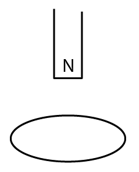Solution:

Average induced emf in time interval Δt = 0.5 s

ε = -( φ2 – φ1)/Δt = -1 V

The induced current is anti-clockwise when seen from the side of the magnet.

Question 15: A wire-loop confined in a plane is rotated in its own plane with some angular velocity. A uniform magnetic field exists in the region. Find the emf induced in the loop.

Solution:

When the wire rotates in its own plane in uniform magnetic field, the magnetic flux remains same. Hence, the flux passing through the loop remains constant and is zero.

Question 16: Figure shows a square loop of side 5 cm being moved towards right at a constant speed of 1 cm/s. The front edge enters the 20 cm wide magnetic field at t = 0. Find the emf induced in the loop at

(a) t = 2s, (b) t = 10 s

(c) t = 22 s, (d) t = 30s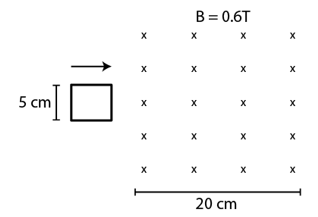Solution:

(a) At t = 2s

Distance moved by coil = 2 x 1 = 2 cm = 2 x 10-2 m

Area under the magnetic field at t = A = 2 x 5 x 10-4 m2

In this case, initial magnetic flux, φ1 = 0 and final magnetic flux, φ2 = BA = 0.6 x (10×10-4) T-m2

Now,

Induced emf in the coil = ε = Δφ/Δt = [0.6 x (10×10-4)]/2

=> ε = 3 x 10-4 V

[Where Δφ = φ2 – φ1]

(b) At t = 10 s

Distance travelled by coil = 10 x 1 = 10 cm

In this case induced emf at this time is zero. Since square loop is completely inside the magnetic field, so no change in flux linked with coil with this time.

(c) At t = 22 s:

Distance moved by coil = 22 x 1 = 22 cm = 22 x 10-2 m

At this time loop is moving out of the field.

In this case, initial magnetic flux, φ1 = 0.6 x (3x5x10-4) T-m and final magnetic flux. φ2 = 0.6 x (3x5x10-4) T-m

Now,

Induced emf in the coil = ε = Δφ/Δt = [-6 x10-4]/2

=> ε = -3 x 10-4 V

[Where Δφ = φ2 – φ1 = -6 x 10-4 T-m2]

(d) At t = 30 s:

At this time, square loop is completely outside the magnetic field and the area of loop through which flux passes is zero.

Question 17: Find the total heat produced in the loop of the previous problem during the interval 0 to 30 s if the resistance of the loop is 4.5 mΩ.

Solution:

Resistance of the loop = 4.5 mΩ = 4.5 x 10-3 Ω

Total heat is the sum of heat produced in different time intervals.

Let,

Heat produced during time interval 0-5s = Ha

Heat produced during time interval 5-20s = Hb

Heat produced during time interval 20-25s = Hc

Heat produced during time interval 25-30s = Hd

=> HT = Ha + Hb + Hc + Hd

In time interval 0-5s emf produced in the loop:

ε = 3x 10-4 V

Current in the coil = i = ε/R = 3 x 10-4/(4.5×10-3)2 = 6.7 x 10-2 A

Heat produced in the coil = Ha = i2Rt

= (6.7 x 10-2)2 x 4.5 x 10-3 x 5

Emf induced in the time interval 5-20s = 0 and

Emf induced in the time interval 25-30s = 0

Current in the coil during this time = i = 0

Heat produced in the coil is also zero.

So Hb = Hd = 0

emf induced in the time interval 20-25s = emf induced at 5s

i.e. ε = 3x 10-4 V

So, the current and heat produced during this interval is same:

i.e. Hc = (6.7 x 10-2)2 x 4.5 x 10-3 x 5

Total heat in the loop during interval 0-30s = 2 x (6.7 x 10-2)2 x 4.5 x 10-3 x 5 = 2 x 10-4 J

Question 18: A uniform magnetic field B exists in a cylindrical region of radius 10 cm as shown in figure. A uniform wire of length 80 cm and resistance 4.0Ω is bent into a square frame and is placed with one side along a diameter of the cylindrical region. If the magnetic field increases at a constant rate of 0.010 T/s, find the current induced in the frame.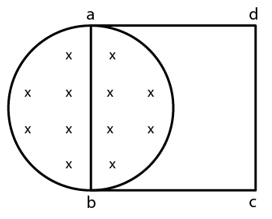Solution:

Area of loop inside magnetic field is equal to the area of semicircle of radius 10 cm

=> A = π r2

The change in magnetic flux is

dφ/dt = (dB/dt ) A = A . dB/dt

The induced emf is:

ε = – dφ/dt = – A . dB/dt

And, current through the loop = i = ε/R = – (A/R) . dB/dt

Substituting the values, we have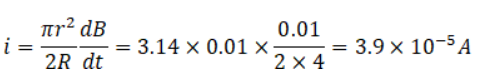Question 19: The magnetic field in the cylindrical region shown in figure increases at a constant rate of 20.0 mT/s. Each side of the square loop abcd and defa has a length of 1.00 cm and a resistance of 4.00 Ω. Find the current (magnitude and since) in the wire ad if

(a) the switch S1 is closed but S2 is open,

(b) S1 is open but S2 is closed,

(c) both S1 and S2 are open and

(d) both S1 and S2 are closed.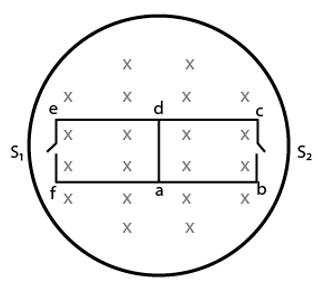Solution:

We know, current through the loop (i) of resistance R = i = -(A/R) dB/dt …(1)

(a) when switch S1 is closed but S2 is open

There is no current flows through loop abcd.

So, net resistance of the loop adef R = 4× 4 = 16 Ω

A = Area of loop adef = 10-4 m2

Here A = 10-4 and dB/dt = 0.02

(1)=> i = 1.25 x 10-7 along ad

(b) S1 is open but S2 is closed

Net resistance of loop abcd = R = 4× 4=16Ω

(1)=> i = (20×10-6)/16 = 1.25 x 10-7 A along da.

(c) When both S1 and S2 is open

No current flows. Hence current in wire is zero.

(d) When both S1 and S2 is closed

The circuit forms a balanced Wheatstone Bridge and no current flows through the wire ad.

Question 20: Figure shows a circular coil of N turns and radius a, connected to a battery of emf ϵ through a rheostat. The rheostat has a total length L and resistance R. The resistance of the coil is r. A small circular loop of radius a’ and resistance r’ is placed coaxially with the coil. The center of the loop is at a distance x from the center of the coil. In the beginning, the sliding contact of the rheostat is at the left end and then onwards it is moved towards right at a constant speed v. Find the emf induced in the small circular loop at the instant

(a) the contact begins to slide and

(b) it has slid through half the length of the rheostat.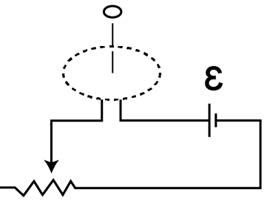Solution: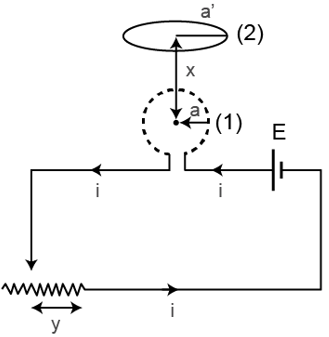The magnetic field due to coil (1) at the center of coil (2):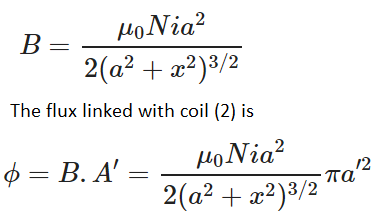Where, In coil (1)

N = Number of turns in coil

i = current in coil

x = distance of center of coil (2) from center of coil (1)

When the sliding contact is at a distance “y” from its right end then the resistance of the rheostat, say R’ is

R’ = (R/L) y

Where, R = Total resistance of rheostat.

The current flowing through the circuit is

i = ε/(R’+r)

Where ϵ is the emf of battery and r is the resistance of the coil.

And magnitude of the emf induced,

ε = d φ/dt